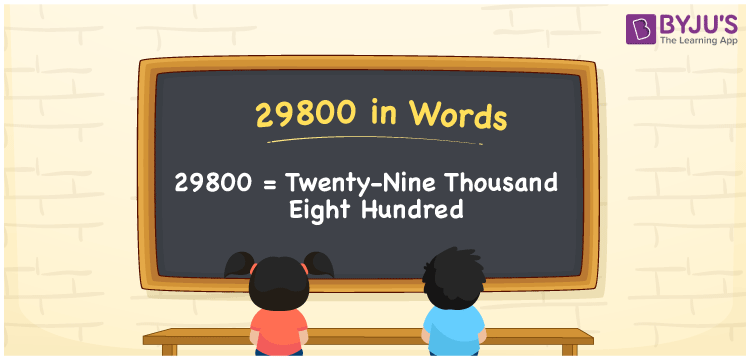# 29800 in words

29800 in words is written as Twenty Nine Thousand Eight Hundred. In 29800, 2 has a place value of ten thousand, 9 is in the place value of thousand and 8 is in the place value of hundred. The article on Place Value gives more information. The number 29800 is used in expressions that relate to money, distance, social media views, and many more. For example, “A newly released song hit Twenty Nine Thousand Eight Hundred views in the first few hours”.

 29800 in words Twenty Nine Thousand Eight Hundred Twenty Nine Thousand Eight Hundred in Numbers 29800

## 29800 in English Words## How to Write 29800 in Words?

We can convert 29800 to words using a place value chart. The number 29800 has 5 digits, so let’s make a chart that shows the place value up to 5 digits.

 Ten thousand Thousands Hundreds Tens Ones 2 9 8 0 0

Thus, we can write the expanded form as:

2 × Ten thousand + 9 × Thousand + 8 × Hundred + 0 × Ten + 0 × One

= 2 × 10000 + 9 × 1000 + 8 × 100 + 0 × 10 + 0 × 1

= 29800.

= Twenty Nine Thousand Eight Hundred.

29800 is the natural number that is succeeded by 29799 and preceded by 29801.

29800 in words – Twenty Nine Thousand Eight Hundred.

Is 29800 an odd number? – No.

Is 29800 an even number? – Yes.

Is 29800 a perfect square number? – No.

Is 29800 a perfect cube number? – No.

Is 29800 a prime number? – No.

Is 29800 a composite number? – Yes.

## Solved Example

1. Write the number 29800 in expanded form

Solution: 2 x 10000 + 9 x 1000 + 8 x 100 + 0 x 10 + 0 x 1

Or Just 2 x 10000 + 9 x 1000 + 8 x 100

We can write 29800 = 20000 + 9000 + 800 + 0 + 0

= 2 x 10000 + 9 x 1000 + 8 x 100 + 0 x 10 + 0 x 1.

## Frequently Asked Questions on 29800 in words

### How to write the number 29800 in words?

29800 in words is written as Twenty Nine Thousand Eight Hundred.

### Is 29800 divisible by 3?

No. 29800 is not divisible by 3.

### Is 29800 divisible by 10?

Yes. 29800 is divisible by 10.